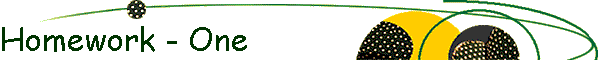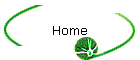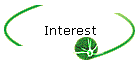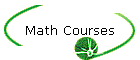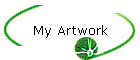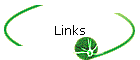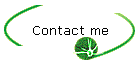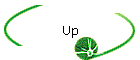College Algebra and Trigonometry II Note: This page contains links to solutions of problems that are similar to the homework problems. Most of the problems are selected from the textbook. They are usually the ones that precede a homework problem (thus, the name: HW-One). The goal is to give the student a close example to help him solve the homework assignment. If the homework problem and the one preceding it are not similar, another problem is created and a (+) sign is added to the number of the problem. Chapter              Format      4           HTML           Mcad      5           HTML           Mcad      6           HTML           Mcad      7           HTML           Mcad      8           HTML           Mcad      9           HTML           Mcad      10         HTML           Mcad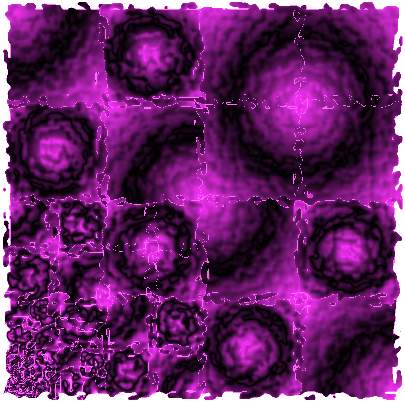Fractals (A. Farhat 2001)